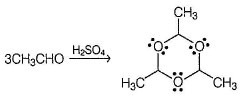Courses

# Test: Keto-Enol Tautomerism And Related Reactions

## 26 Questions MCQ Test Chemistry for JEE | Test: Keto-Enol Tautomerism And Related Reactions

Description
This mock test of Test: Keto-Enol Tautomerism And Related Reactions for Class 12 helps you for every Class 12 entrance exam. This contains 26 Multiple Choice Questions for Class 12 Test: Keto-Enol Tautomerism And Related Reactions (mcq) to study with solutions a complete question bank. The solved questions answers in this Test: Keto-Enol Tautomerism And Related Reactions quiz give you a good mix of easy questions and tough questions. Class 12 students definitely take this Test: Keto-Enol Tautomerism And Related Reactions exercise for a better result in the exam. You can find other Test: Keto-Enol Tautomerism And Related Reactions extra questions, long questions & short questions for Class 12 on EduRev as well by searching above.
QUESTION: 1

### Only One Option Correct Type Direction (Q. Nos. 1-14) This section contains 14 multiple choice questions. Each question has four choices (a), (b), (c) and (d), out of which ONLY ONE is correct. Q.  In hexane-2,4-dione, how many different mono-enols are possible?

Solution: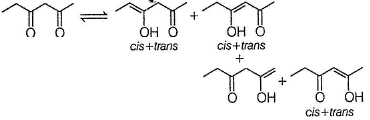QUESTION: 2

### What is the correct order of equilibrium enol content of the following compounds? I. CH3COCH3 II. CH3COCH2COOC2H5 III. CH3COCH2COCH3 IV. CH3COCH2COH

Solution:

A 1,3-diketo compound forms more stable enol than a monocarbonyls. Also ester group forms less stable enol than carbonyls. Hence, III, a 1 , 3-diketo ne form s highest enol content while I (monocarbonyl) forms least enol content at equilibrium.

QUESTION: 3

### In which of the following compounds, enol form exist?

Solution:

Both option (a) and option (c) forms enol but option (b) does not form enol.

QUESTION: 4

Among the following compounds, one that will not show keto-enol tautomerism is

Solution:

sp2 hybridisation is very less stable at bridgehead carbon of a bicyclic compound.

QUESTION: 5

Arrange the following in the increasing order of hydrate content at equilibrium in aqueous solution

I. H2CO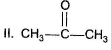III. C6H5CHO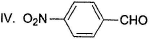Solution:

Due to negligible steric hindrance, form aldehyde form s large hydrate content at equilibrium. Also electron withdrawing group in (IV) increases hydrate content compared to III.

QUESTION: 6

For which of the following equilibrium, K< 1 ?

Solution:

In the absence of any special stability factor, a hydrate of ketone is highly unstable.

QUESTION: 7

Arrange the following in the increasing order of stability of their most stable enol.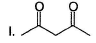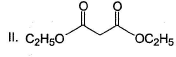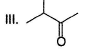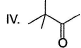Solution:

Diketo (I) forms highest enol content due to stabilisation of enol by intermolecular H-bonding. Electron donating resonance effect by ester group slightly decreases enol content.

QUESTION: 8

Which of the following observation does not establish the existence of keto-enol tautomerism in acetone?

Solution: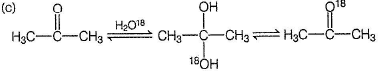The above exchange occur due to existence of hydrate equilibrium.

QUESTION: 9

Hemiacetals are usually unstable whereas acetals are stable. Which has the most stable hemiacetal?

Solution: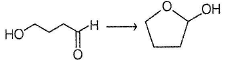If a hemiacetal is five or six membered cyclic, they are stable.

QUESTION: 10

Which form s most stable acetal in the given condition?

Solution: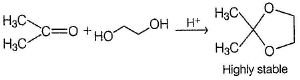QUESTION: 11

Consider the following reaction,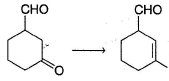Q.

The appropriatensequence of reagent that can best bring about the above conversion is

Solution: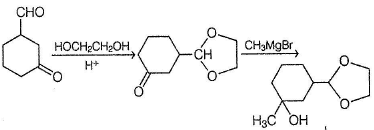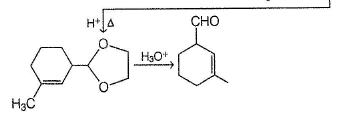QUESTION: 12

What is the major product in the following reaction?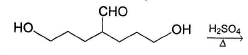Solution:

Acetal is form ed by cyclisation

QUESTION: 13

Which of the following has greater enol content than keto counter part?

Solution:

It is 1, 3-dik eto compound, forms stable enol.

QUESTION: 14

Arrange the following in the increasing order of acidic strength

I. H2CO
II. CH3CHO
III. C6H5CH2CHO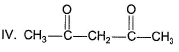Solution:

IV is most acidic as its conjugate base is resonance stabilised by two carbonyl groups, followed by III whose conjugate base is resonance stabilised by a carbonyl group and phenyi ring.

*Multiple options can be correct
QUESTION: 15

One or More than One Options Correct Type

Direction (Q. Nos. 15-19) This section contains 5 multiple choice questions. Each question has four choices (a), (b), (c) and (d), out of which ONE or MORE THAN ONE are correct.

Q.

Which of the following has (have) more than one enol tautomers?

Solution: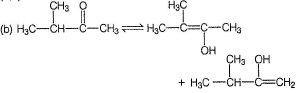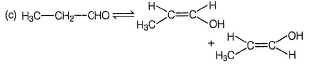*Multiple options can be correct
QUESTION: 16

Which can be deduced correctly regarding keto-enol tautomerism in general?

Solution:

Increasing temperature increases equilibrium content of less stable enol tautomers.
Enolisation decreases stability, hence introducing two or more enol groups are further more difficult.
Enols of ketones are more substituted at double bond, hence more stable.
Both acid and base catalyses keto-enol tautomerism.

*Multiple options can be correct
QUESTION: 17

The correct statement(s) regarding hydrates of aldehyde and ketone is/are

Solution:

Hydrates of aldehydes and ketones are less stable than anhydrous form (gem diols are unstable).
Hydrates form H-bonds with water, hence hydrate content is more in water than in hexane.
This exchange occur via hydrate.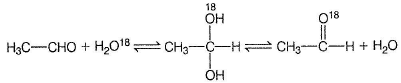*Multiple options can be correct
QUESTION: 18

For which of the following equilibrium, Kc is greater than 1?

Solution:

Five and six-membered cyclic hemiacetals are more stable than its hydroxy aldehydes/ketones, predominate at equilibrium. Cyclic acetals are highly stable, predominate at equilibrium.

*Multiple options can be correct
QUESTION: 19

Consider the reaction below,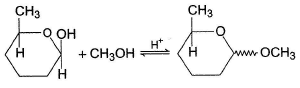The correct statement(s) regarding the above process is/are

Solution:

Product is an acetal, more stable than hemiacetal reactant. Acetal is formed via carbocation, both diastereomers are formed.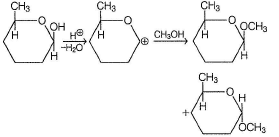QUESTION: 20

Comprehension Type

Direction (Q. Nos. 20-22) This section contains a paragraph, describing theory, experiments, data, etc. Three questions related to the paragraph have been given. Each question has only one correct answer among the four given options (a), (b), (c) and (d).

Passage

In general, ketones are less reactive than aldehydes in nucleophilic addition reaction, for both steric and electronic reasons.
Hence, if a keto-aldehyde is treated with nucleophilic reagent, reaction occur first at aldehyde group.

Q.

The major final product in the following reaction is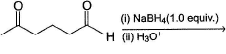Solution: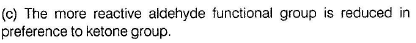The more reactive aldehyde functional group is reduced in preference to ketone group.

QUESTION: 21

In general, ketones are less reactive than aldehydes in nucleophilic addition reaction, for both steric and electronic reasons.
Hence, if a keto-aldehyde is treated with nucleophilic reagent, reaction occur first at aldehyde group.

Q.

How the following transformation can be best brought about?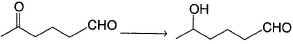Solution:

Aldehyde being more reactive, protected first by acetal formation followed by reduction of ketone group.

QUESTION: 22

In general, ketones are less reactive than aldehydes in nucleophilic addition reaction, for both steric and electronic reasons.
Hence, if a keto-aldehyde is treated with nucleophilic reagent, reaction occur first at aldehyde group.

Consider the following reaction,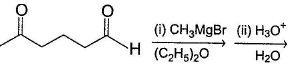Q.

Major product is

Solution: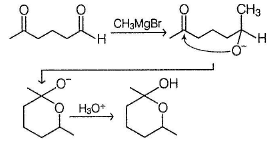*Answer can only contain numeric values
QUESTION: 23

One Integer Value Correct Type

Direction (Q. Nos. 23-26) This section contains 4 questions. When worked out will result in an integer from 0 to 9 (both inclusive).

Q.

How many different enols exist for 4-methyl-3- hexanone ?

Solution: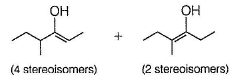*Answer can only contain numeric values
QUESTION: 24

If all the mono and dienols are considered, how many enols are possible for 2,4-pentanedione?

Solution: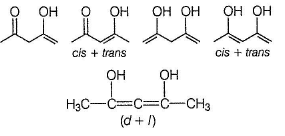*Answer can only contain numeric values
QUESTION: 25

If butanone is treated with D2O18/DCI, isotopic exchange occur. What maximum gain in molecular mass is possible in the present case?

Solution: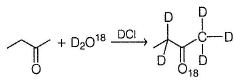Gain of 7 units in molar mass is observed, five units due to 'D' and tw o units due to `O18'.

*Answer can only contain numeric values
QUESTION: 26

When acetaldehyde is treated with catalytic amount of H2SO4, a stable association product X is formed. How many lone pair of electrons are present in XI

Solution: